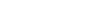# Enterprise Wireless Vibration Sensor Data Packet Clarification

#1

Im trying to understand the documentation, here.

For the time series data, Sample 1, X - X - Y - Y - Z - Z , what does that actually mean?

Do I concatenate/subtract the X and X values to get the X value for sample 1, then divide that by 2048? or do I divide each of those bytes by 2048 then concatenate/subtract the values?

Would like clarification in deciphering the documentation please. Thank you!

#2

Hi Sufyan,
The conversion works the same way as shown in Table 3 for Run mode. However, in this case, you have only two bytes therefore it will be converted to count value using the following formula
(X << 8)+ X
The resultant count value will be divided by 2048 to get acceleration in mg units.